# ahrsfilter

Orientation from accelerometer, gyroscope, and magnetometer readings

## Description

The ahrsfilter System object™ fuses accelerometer, magnetometer, and gyroscope sensor data to estimate device orientation.

To estimate device orientation:

1. Create the ahrsfilter object and set its properties.

2. Call the object with arguments, as if it were a function.

## Creation

### Syntax

FUSE = ahrsfilter
FUSE = ahrsfilter('ReferenceFrame',RF)
FUSE = ahrsfilter(___,Name,Value)

### Description

example

FUSE = ahrsfilter returns an indirect Kalman filter System object, FUSE, for sensor fusion of accelerometer, gyroscope, and magnetometer data to estimate device orientation and angular velocity. The filter uses a 12-element state vector to track the estimation error for the orientation, the gyroscope bias, the linear acceleration, and the magnetic disturbance.
FUSE = ahrsfilter('ReferenceFrame',RF) returns an ahrsfilter System object that fuses accelerometer, gyroscope, and magnetometer data to estimate device orientation relative to the reference frame RF. Specify RF as 'NED' (North-East-Down) or 'ENU' (East-North-Up). The default value is 'NED'.

example

FUSE = ahrsfilter(___,Name,Value) sets each property Name to the specified Value. Unspecified properties have default values.

## Properties

expand all

Unless otherwise indicated, properties are nontunable, which means you cannot change their values after calling the object. Objects lock when you call them, and the release function unlocks them.

If a property is tunable, you can change its value at any time.

Input sample rate of the sensor data in Hz, specified as a positive scalar.

Tunable: No

Data Types: single | double

Decimation factor by which to reduce the input sensor data rate as part of the fusion algorithm, specified as a positive integer.

The number of rows of the inputs –– accelReadings, gyroReadings, and magReadings –– must be a multiple of the decimation factor.

Data Types: single | double

Variance of accelerometer signal noise in (m/s2)2, specified as a positive real scalar.

Tunable: Yes

Data Types: single | double

Variance of magnetometer signal noise in μT2, specified as a positive real scalar.

Tunable: Yes

Data Types: single | double

Variance of gyroscope signal noise in (rad/s)2, specified as a positive real scalar.

Tunable: Yes

Data Types: single | double

Variance of gyroscope offset drift in (rad/s)2, specified as a positive real scalar.

Tunable: Yes

Data Types: single | double

Variance of linear acceleration noise in (m/s2)2, specified as a positive real scalar. Linear acceleration is modeled as a lowpass-filtered white noise process.

Tunable: Yes

Data Types: single | double

Decay factor for linear acceleration drift, specified as a scalar in the range [0,1). If linear acceleration is changing quickly, set LinearAcclerationDecayFactor to a lower value. If linear acceleration changes slowly, set LinearAcclerationDecayFactor to a higher value. Linear acceleration drift is modeled as a lowpass-filtered white noise process.

Tunable: Yes

Data Types: single | double

Variance of magnetic disturbance noise in μT2, specified as a real finite positive scalar.

Tunable: Yes

Data Types: single | double

Decay factor for magnetic disturbance, specified as a positive scalar in the range [0,1]. Magnetic disturbance is modeled as a first order Markov process.

Tunable: Yes

Data Types: single | double

Covariance matrix for process noise, specified as a 12-by-12 matrix. The default is:

 Columns 1 through 6 0.000006092348396 0 0 0 0 0 0 0.000006092348396 0 0 0 0 0 0 0.000006092348396 0 0 0 0 0 0 0.000076154354947 0 0 0 0 0 0 0.000076154354947 0 0 0 0 0 0 0.000076154354947 0 0 0 0 0 0 0 0 0 0 0 0 0 0 0 0 0 0 0 0 0 0 0 0 0 0 0 0 0 0 0 0 0 0 0 0 Columns 7 through 12 0 0 0 0 0 0 0 0 0 0 0 0 0 0 0 0 0 0 0 0 0 0 0 0 0 0 0 0 0 0 0 0 0 0 0 0 0.009623610000000 0 0 0 0 0 0 0.009623610000000 0 0 0 0 0 0 0.009623610000000 0 0 0 0 0 0 0.600000000000000 0 0 0 0 0 0 0.600000000000000 0 0 0 0 0 0 0.600000000000000

The initial process covariance matrix accounts for the error in the process model.

Data Types: single | double

Expected estimate of magnetic field strength in μT, specified as a real positive scalar. The expected magnetic field strength is an estimate of the magnetic field strength of the Earth at the current location.

Tunable: Yes

Data Types: single | double

Output orientation format, specified as 'quaternion' or 'Rotation matrix'. The size of the output depends on the input size, N, and the output orientation format:

• 'quaternion' –– Output is an N-by-1 quaternion (Sensor Fusion and Tracking Toolbox).

• 'Rotation matrix' –– Output is a 3-by-3-by-N rotation matrix.

Data Types: char | string

## Usage

### Syntax

[orientation,angularVelocity] = FUSE(accelReadings,gyroReadings,magReadings)

### Description

example

[orientation,angularVelocity] = FUSE(accelReadings,gyroReadings,magReadings) fuses accelerometer, gyroscope, and magnetometer data to compute orientation and angular velocity measurements. The algorithm assumes that the device is stationary before the first call.

### Input Arguments

expand all

Accelerometer readings in the sensor body coordinate system in m/s2, specified as an N-by-3 matrix. N is the number of samples, and the three columns of accelReadings represent the [x y z] measurements. Accelerometer readings are assumed to correspond to the sample rate specified by the SampleRate (Sensor Fusion and Tracking Toolbox) property.

Data Types: single | double

Gyroscope readings in the sensor body coordinate system in rad/s, specified as an N-by-3 matrix. N is the number of samples, and the three columns of gyroReadings represent the [x y z] measurements. Gyroscope readings are assumed to correspond to the sample rate specified by the SampleRate (Sensor Fusion and Tracking Toolbox) property.

Data Types: single | double

Magnetometer readings in the sensor body coordinate system in µT, specified as an N-by-3 matrix. N is the number of samples, and the three columns of magReadings represent the [x y z] measurements. Magnetometer readings are assumed to correspond to the sample rate specified by the SampleRate (Sensor Fusion and Tracking Toolbox) property.

Data Types: single | double

### Output Arguments

expand all

Orientation that can rotate quantities from the local navigation coordinate system to a body coordinate system, returned as quaternions or an array. The size and type of orientation depends on whether the OrientationFormat (Sensor Fusion and Tracking Toolbox) property is set to 'quaternion' or 'Rotation matrix':

• 'quaternion' –– the output is an M-by-1 vector of quaternions, with the same underlying data type as the inputs

• 'Rotation matrix' –– the output is a 3-by-3-by-M array of rotation matrices the same data type as the inputs

The number of input samples, N, and the DecimationFactor (Sensor Fusion and Tracking Toolbox) property determine M.

You can use orientation in a rotateframe (Sensor Fusion and Tracking Toolbox) function to rotate quantities from a local navigation system to a sensor body coordinate system.

Data Types: quaternion | single | double

Angular velocity with gyroscope bias removed in the sensor body coordinate system in rad/s, returned as an M-by-3 array. The number of input samples, N, and the DecimationFactor property determine M.

Data Types: single | double

## Object Functions

To use an object function, specify the System object as the first input argument. For example, to release system resources of a System object named obj, use this syntax:

release(obj)

expand all

 tune Tune ahrsfilter parameters to reduce estimation error
 step Run System object algorithm release Release resources and allow changes to System object property values and input characteristics reset Reset internal states of System object

## Examples

collapse all

Load the rpy_9axis file, which contains recorded accelerometer, gyroscope, and magnetometer sensor data from a device oscillating in pitch (around y-axis), then yaw (around z-axis), and then roll (around x-axis). The file also contains the sample rate of the recording.

load 'rpy_9axis' sensorData Fs accelerometerReadings = sensorData.Acceleration; gyroscopeReadings = sensorData.AngularVelocity; magnetometerReadings = sensorData.MagneticField;

Create an ahrsfilter System object™ with SampleRate set to the sample rate of the sensor data. Specify a decimation factor of two to reduce the computational cost of the algorithm.

decim = 2; fuse = ahrsfilter('SampleRate',Fs,'DecimationFactor',decim);

Pass the accelerometer readings, gyroscope readings, and magnetometer readings to the ahrsfilter object, fuse, to output an estimate of the sensor body orientation over time. By default, the orientation is output as a vector of quaternions.

q = fuse(accelerometerReadings,gyroscopeReadings,magnetometerReadings);

Orientation is defined by angular displacement required to rotate a parent coordinate system to a child coordinate system. Plot the orientation in Euler angles in degrees over time.

ahrsfilter correctly estimates the change in orientation over time, including the south-facing initial orientation.

time = (0:decim:size(accelerometerReadings,1)-1)/Fs; plot(time,eulerd(q,'ZYX','frame')) title('Orientation Estimate') legend('z-axis', 'y-axis', 'x-axis') ylabel('Rotation (degrees)')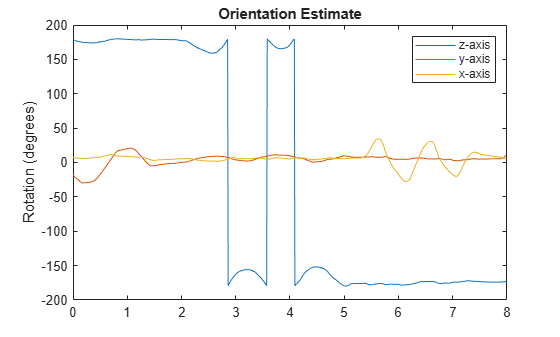This example shows how performance of the ahrsfilter System object™ is affected by magnetic jamming.

Load StationaryIMUReadings, which contains accelerometer, magnetometer, and gyroscope readings from a stationary IMU.

load 'StationaryIMUReadings.mat' accelReadings magReadings gyroReadings SampleRate numSamples = size(accelReadings,1); 

The ahrsfilter uses magnetic field strength to stabilize its orientation against the assumed constant magnetic field of the Earth. However, there are many natural and man-made objects which output magnetic fields and can confuse the algorithm. To account for the presence of transient magnetic fields, you can set the MagneticDisturbanceNoise property on the ahrsfilter object.

Create an ahrsfilter object with the decimation factor set to 2 and note the default expected magnetic field strength.

decim = 2; FUSE = ahrsfilter('SampleRate',SampleRate,'DecimationFactor',decim); 

Fuse the IMU readings using the attitude and heading reference system (AHRS) filter, and then visualize the orientation of the sensor body over time. The orientation fluctuates at the beginning and stabilizes after approximately 60 seconds.

orientation = FUSE(accelReadings,gyroReadings,magReadings); orientationEulerAngles = eulerd(orientation,'ZYX','frame'); time = (0:decim:(numSamples-1))'/SampleRate; figure(1) plot(time,orientationEulerAngles(:,1), ... time,orientationEulerAngles(:,2), ... time,orientationEulerAngles(:,3)) xlabel('Time (s)') ylabel('Rotation (degrees)') legend('z-axis','y-axis','x-axis') title('Filtered IMU Data')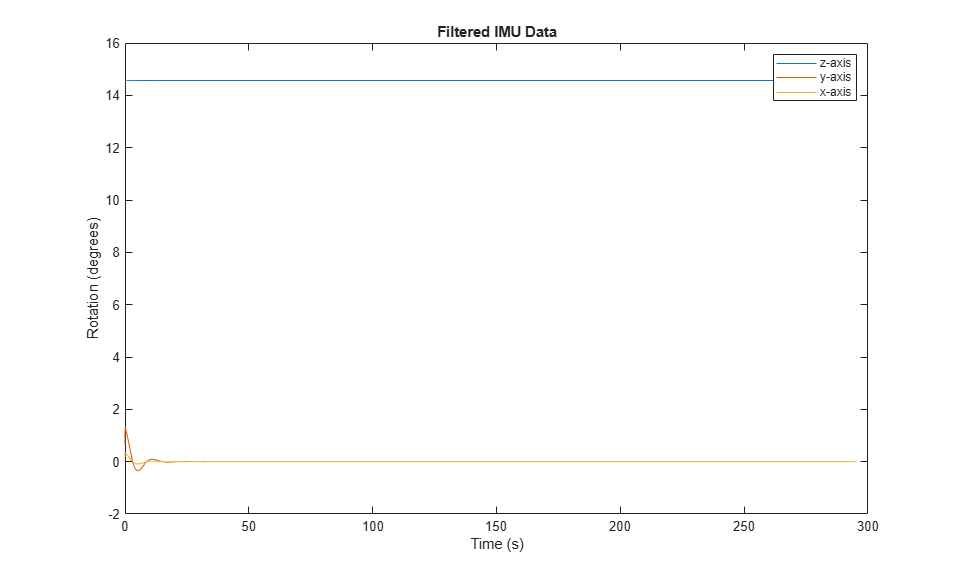Mimic magnetic jamming by adding a transient, strong magnetic field to the magnetic field recorded in the magReadings. Visualize the magnetic field jamming.

jamStrength = [10,5,2]; startStop = (50*SampleRate):(150*SampleRate); jam = zeros(size(magReadings)); jam(startStop,:) = jamStrength.*ones(numel(startStop),3); magReadings = magReadings + jam; figure(2) plot(time,magReadings(1:decim:end,:)) xlabel('Time (s)') ylabel('Magnetic Field Strength (\mu T)') title('Simulated Magnetic Field with Jamming') legend('z-axis','y-axis','x-axis')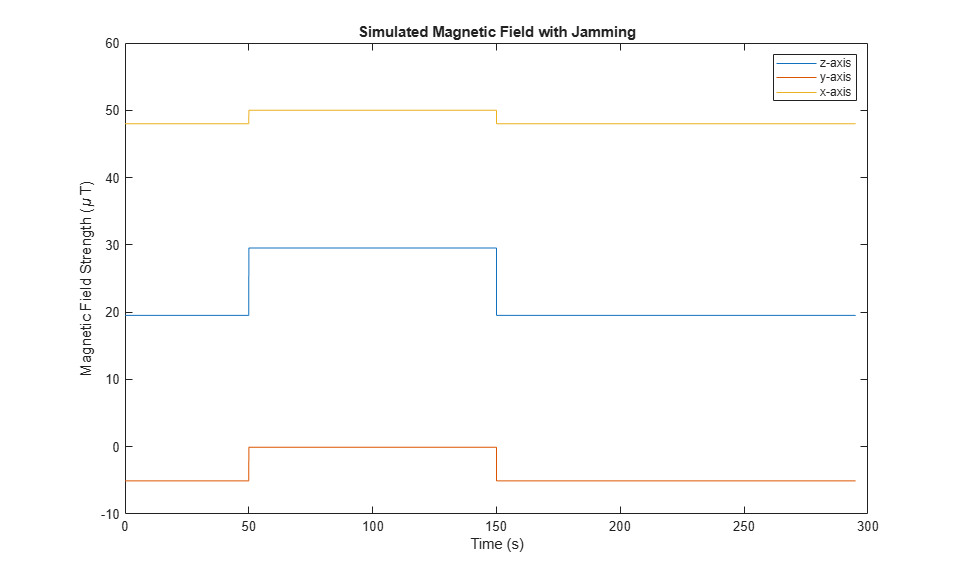Run the simulation again using the magReadings with magnetic jamming. Plot the results and note the decreased performance in orientation estimation.

reset(FUSE) orientation = FUSE(accelReadings,gyroReadings,magReadings); orientationEulerAngles = eulerd(orientation,'ZYX','frame'); figure(3) plot(time,orientationEulerAngles(:,1), ... time,orientationEulerAngles(:,2), ... time,orientationEulerAngles(:,3)) xlabel('Time (s)') ylabel('Rotation (degrees)') legend('z-axis','y-axis','x-axis') title('Filtered IMU Data with Magnetic Disturbance and Default Properties')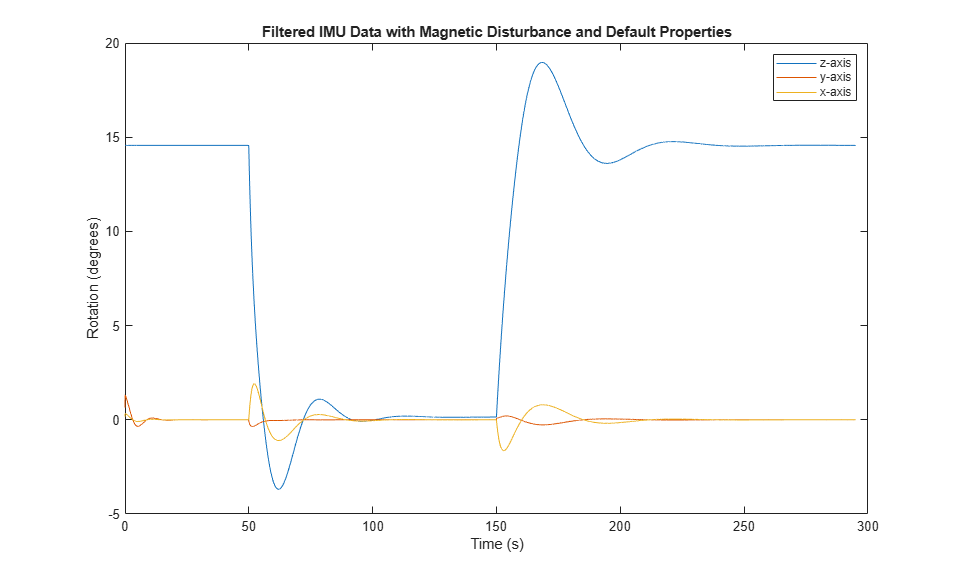The magnetic jamming was misinterpreted by the AHRS filter, and the sensor body orientation was incorrectly estimated. You can compensate for jamming by increasing the MagneticDisturbanceNoise property. Increasing the MagneticDisturbanceNoise property increases the assumed noise range for magnetic disturbance, and the entire magnetometer signal is weighted less in the underlying fusion algorithm of ahrsfilter.

Set the MagneticDisturbanceNoise to 200 and run the simulation again.

The orientation estimation output from ahrsfilter is more accurate and less affected by the magnetic transient. However, because the magnetometer signal is weighted less in the underlying fusion algorithm, the algorithm may take more time to restabilize.

reset(FUSE) FUSE.MagneticDisturbanceNoise = 20; orientation = FUSE(accelReadings,gyroReadings,magReadings); orientationEulerAngles = eulerd(orientation,'ZYX','frame'); figure(4) plot(time,orientationEulerAngles(:,1), ... time,orientationEulerAngles(:,2), ... time,orientationEulerAngles(:,3)) xlabel('Time (s)') ylabel('Rotation (degrees)') legend('z-axis','y-axis','x-axis') title('Filtered IMU Data with Magnetic Disturbance and Modified Properties')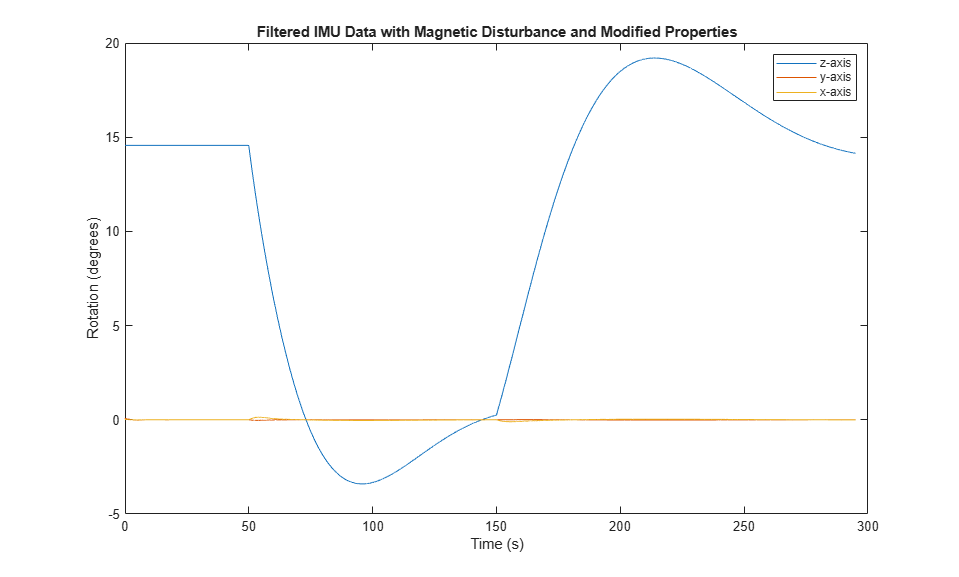This example uses the ahrsfilter System object™ to fuse 9-axis IMU data from a sensor body that is shaken. Plot the quaternion distance between the object and its final resting position to visualize performance and how quickly the filter converges to the correct resting position. Then tune parameters of the ahrsfilter so that the filter converges more quickly to the ground-truth resting position.

Load IMUReadingsShaken into your current workspace. This data was recorded from an IMU that was shaken then laid in a resting position. Visualize the acceleration, magnetic field, and angular velocity as recorded by the sensors.

load 'IMUReadingsShaken' accelReadings gyroReadings magReadings SampleRate numSamples = size(accelReadings,1); time = (0:(numSamples-1))'/SampleRate; figure(1) subplot(3,1,1) plot(time,accelReadings) title('Accelerometer Reading') ylabel('Acceleration (m/s^2)') subplot(3,1,2) plot(time,magReadings) title('Magnetometer Reading') ylabel('Magnetic Field (\muT)') subplot(3,1,3) plot(time,gyroReadings) title('Gyroscope Reading') ylabel('Angular Velocity (rad/s)') xlabel('Time (s)')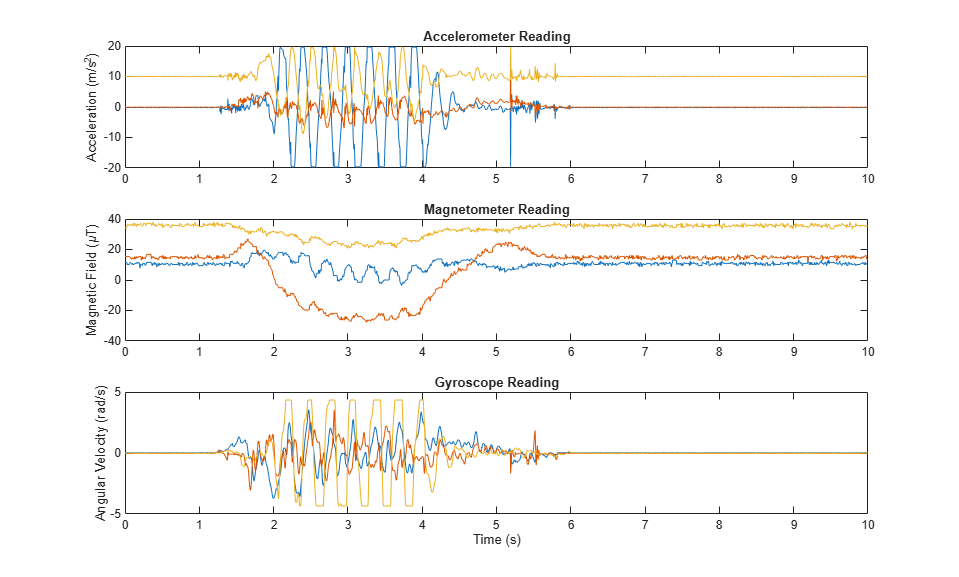Create an ahrsfilter and then fuse the IMU data to determine orientation. The orientation is returned as a vector of quaternions; convert the quaternions to Euler angles in degrees. Visualize the orientation of the sensor body over time by plotting the Euler angles required, at each time step, to rotate the global coordinate system to the sensor body coordinate system.

fuse = ahrsfilter('SampleRate',SampleRate); orientation = fuse(accelReadings,gyroReadings,magReadings); orientationEulerAngles = eulerd(orientation,'ZYX','frame'); figure(2) plot(time,orientationEulerAngles(:,1), ... time,orientationEulerAngles(:,2), ... time,orientationEulerAngles(:,3)) xlabel('Time (s)') ylabel('Rotation (degrees)') title('Orientation over Time') legend('Rotation around z-axis', ... 'Rotation around y-axis', ... 'Rotation around x-axis')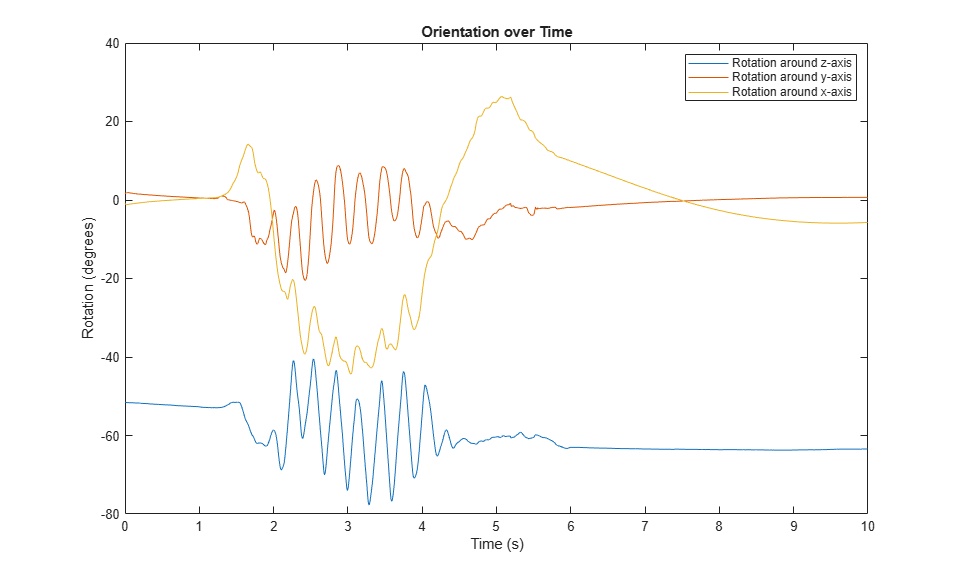In the IMU recording, the shaking stops after approximately six seconds. Determine the resting orientation so that you can characterize how fast the ahrsfilter converges.

To determine the resting orientation, calculate the averages of the magnetic field and acceleration for the final four seconds and then use the ecompass function to fuse the data.

Visualize the quaternion distance from the resting position over time.

restingOrientation = ecompass(mean(accelReadings(6*SampleRate:end,:)), ... mean(magReadings(6*SampleRate:end,:))); figure(3) plot(time,rad2deg(dist(restingOrientation,orientation))) hold on xlabel('Time (s)') ylabel('Quaternion Distance (degrees)')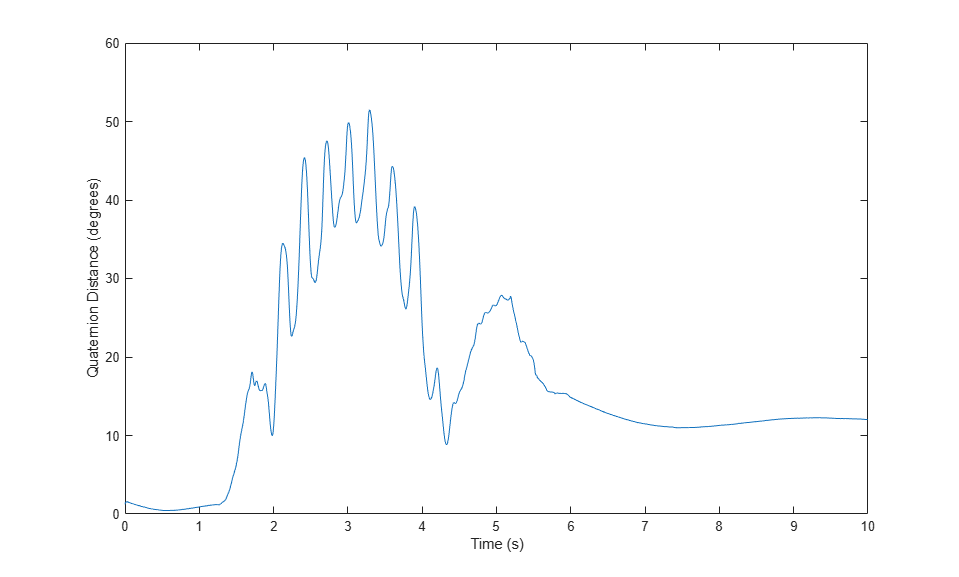Modify the default ahrsfilter properties so that the filter converges to gravity more quickly. Increase the GyroscopeDriftNoise to 1e-2 and decrease the LinearAccelerationNoise to 1e-4. This instructs the ahrsfilter algorithm to weigh gyroscope data less and accelerometer data more. Because the accelerometer data provides the stabilizing and consistent gravity vector, the resulting orientation converges more quickly.

Reset the filter, fuse the data, and plot the results.

fuse.LinearAccelerationNoise = 1e-4; fuse.GyroscopeDriftNoise = 1e-2; reset(fuse) orientation = fuse(accelReadings,gyroReadings,magReadings); figure(3) plot(time,rad2deg(dist(restingOrientation,orientation))) legend('Default AHRS Filter','Tuned AHRS Filter')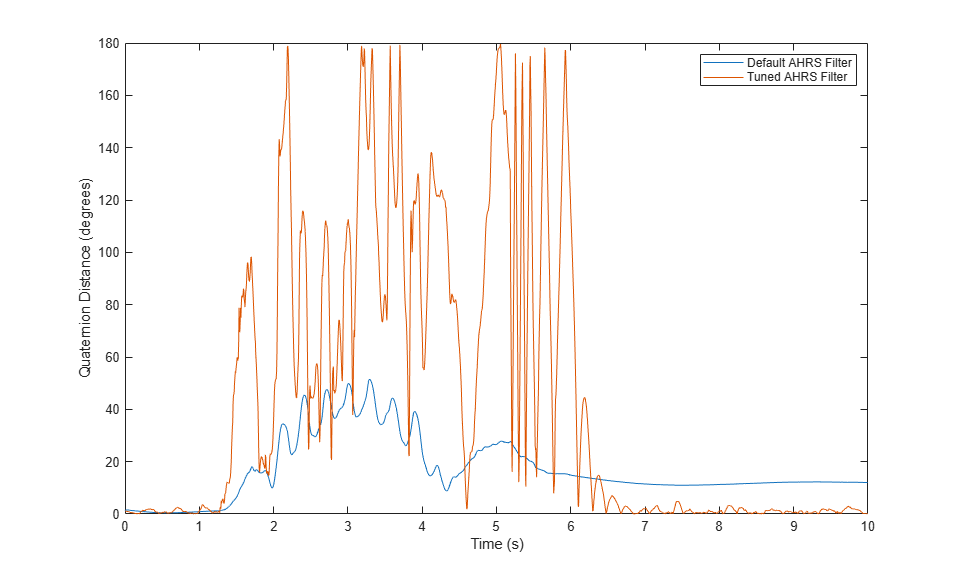## Algorithms

expand all

Note: The following algorithm only applies to an NED reference frame.

The ahrsfilter uses the nine-axis Kalman filter structure described in  (Sensor Fusion and Tracking Toolbox). The algorithm attempts to track the errors in orientation, gyroscope offset, linear acceleration, and magnetic disturbance to output the final orientation and angular velocity. Instead of tracking the orientation directly, the indirect Kalman filter models the error process, x, with a recursive update:

${x}_{k}=\left[\begin{array}{c}{\theta }_{k}\\ {b}_{k}\\ {a}_{k}\\ {d}_{k}\end{array}\right]={F}_{k}\left[\begin{array}{c}{\theta }_{k-1}\\ {b}_{k-1}\\ {a}_{k-1}\\ {d}_{k-1}\end{array}\right]+{w}_{k}$

where xk is a 12-by-1 vector consisting of:

• θk –– 3-by-1 orientation error vector, in degrees, at time k

• bk –– 3-by-1 gyroscope zero angular rate bias vector, in deg/s, at time k

• ak –– 3-by-1 acceleration error vector measured in the sensor frame, in g, at time k

• dk –– 3-by-1 magnetic disturbance error vector measured in the sensor frame, in µT, at time k

and where wk is a 12-by-1 additive noise vector, and Fk is the state transition model.

Because xk is defined as the error process, the a priori estimate is always zero, and therefore the state transition model, Fk, is zero. This insight results in the following reduction of the standard Kalman equations:

Standard Kalman equations:

$\begin{array}{l}{x}_{k}^{-}={F}_{k}{x}_{k-1}^{+}\\ {P}_{k}^{-}={F}_{k}{P}_{k-1}^{+}{F}_{k}^{T}+{Q}_{k}\\ \\ {y}_{k}={z}_{k}-{H}_{k}{x}_{k}^{-}\\ {S}_{k}\text{\hspace{0.17em}}={R}_{k}+{H}_{k}{P}_{k}^{-}{H}_{k}{}^{T}\\ {K}_{k}={P}_{k}^{-}{H}_{k}^{T}{\left({S}_{k}\right)}^{-1}\\ {x}_{k}^{+}={x}_{k}^{-}+{K}_{k}{y}_{k}\\ {P}_{k}^{+}={P}_{k}{}^{-}-{K}_{k}{H}_{k}{P}_{k}^{-}\end{array}$

Kalman equations used in this algorithm:

$\begin{array}{l}{x}_{k}^{-}=0\\ {P}_{k}^{-}={Q}_{k}\\ \\ {y}_{k}={z}_{k}\\ {S}_{k}\text{\hspace{0.17em}}={R}_{k}+{H}_{k}{P}_{k}^{-}{H}_{k}{}^{T}\\ {K}_{k}={P}_{k}^{-}{H}_{k}^{T}{\left({S}_{k}\right)}^{-1}\\ {x}_{k}^{+}={K}_{k}{y}_{k}\\ {P}_{k}^{+}={P}_{k}{}^{-}-{K}_{k}{H}_{k}{P}_{k}^{-}\end{array}$

where:

• xk –– predicted (a priori) state estimate; the error process

• Pk –– predicted (a priori) estimate covariance

• yk –– innovation

• Sk –– innovation covariance

• Kk –– Kalman gain

• xk+ –– updated (a posteriori) state estimate

• Pk+ –– updated (a posteriori) estimate covariance

k represents the iteration, the superscript + represents an a posteriori estimate, and the superscript represents an a priori estimate.

The graphic and following steps describe a single frame-based iteration through the algorithm.Before the first iteration, the accelReadings, gyroReadings, and magReadings inputs are chunked into DecimationFactor-by-3 frames. For each chunk, the algorithm uses the most current accelerometer and magnetometer readings corresponding to the chunk of gyroscope readings.

 Roetenberg, D., H.J. Luinge, C.T.M. Baten, and P.H. Veltink. "Compensation of Magnetic Disturbances Improves Inertial and Magnetic Sensing of Human Body Segment Orientation." IEEE Transactions on Neural Systems and Rehabilitation Engineering. Vol. 13. Issue 3, 2005, pp. 395-405.